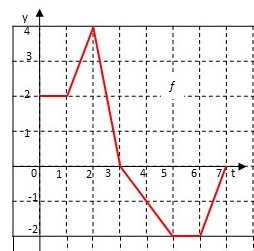# Let g(x) = \int_0^x f(t) dt, where f is the function whose graph is shown. Evaluate: g(0), g(1),...

## Question:

Let {eq}g(x) = \int_0^x f(t) dt, {/eq} where {eq}f {/eq} is the function whose graph is shown.(a) Evaluate: {eq}g(0) {/eq}, {eq}g(1) {/eq}, {eq}g(2) {/eq}, {eq}g(3) {/eq}, and {eq}g(6) {/eq}.

(b) On what interval is {eq}g {/eq} increasing?

(c) Where does {eq}g {/eq} have a maximum value?

## The Area Interpretation of the Integral:

The integral {eq}\int_a^b f(x) \, dx {/eq} can be thought of as the signed area between the graph of {eq}y=f(x) {/eq} and the {eq}x {/eq}-axis for {eq}a \le x \le b {/eq}. By this, we mean that:

{eq}\displaystyle \int_a^b f(x) \, dx = P - N {/eq}

where {eq}P {/eq} is the area below the graph of {eq}y=f(x) {/eq} and above the {eq}x {/eq}-axis, and {eq}N {/eq} is the area below the {eq}x {/eq}-axis and above the graph of {eq}y=f(x) {/eq}.

## Answer and Explanation:

(a) First, we have {eq}g(0)=\int_0^0 f(t) \, dt=0 {/eq}.

To find the other values of {eq}g {/eq}, we count squares. Because the graph of {eq}f {/eq} consists of straight line segments, we can find the area of a triangle under that graph by counting the number of squares in the rectangle with the same base and height and dividing by 2. So we have:

{eq}\begin{align*} g(0)&=0\\ g(1)&=2&\text{(a single rectangle of base 1 and height 2)}\\ g(2)&=5&\text{(adding another rectangle of the same size, along with a triangle of base 1 and height 2)}\\ g(3)&=7&\text{(adding a triangle of base 1 and height 4)}\\ g(6)&=3&\text{(subtracting an area of 5: a triangle of base 2 and height 2, and a rectangle of base 1 and height 2).} \end{align*} {/eq}

(b) The function {eq}g {/eq} is increasing on any interval where {eq}f {/eq} is nonnegative. So {eq}g {/eq} is an increasing function on the interval {eq}\boxed{[0,3]} \, {/eq}.

(c) Since {eq}g {/eq} is increasing on {eq}[0,3] {/eq} and decreasing on {eq}[3,7] {/eq}, {eq}g {/eq} achieves its maximum value at {eq}\boxed{t=3}\, {/eq}.